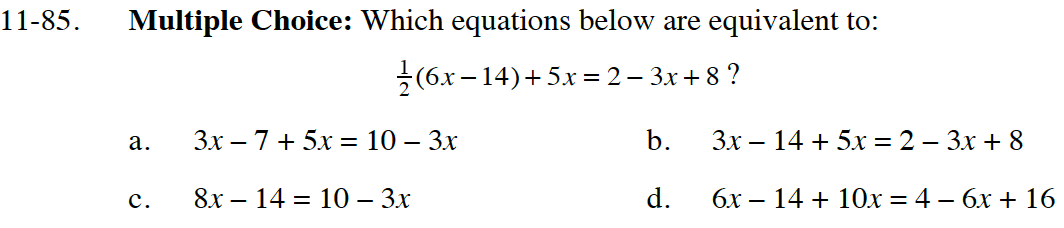### Home > CC4 > Chapter 11 > Lesson 11.2.3 > Problem11-85

11-85.
1. Multiple Choice: Which equations below are equivalent to: Homework Help ✎(6x − 14) + 5x = 2 − 3x + 8?

2.  A. 3x − 7 + 5x = 10 − 3x B. 3x − 14 + 5x = 2 − 3x + 8 C. 8x − 14 = 10 − 3x D. 6x − 14 + 10x = 4 − 6x + 16Try simplifying each of the equations. Which equations are equivalent?